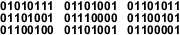Space between bytes.

## What can this tool do?The word 'Wikipedia' represented in ASCII binary.

A binary code represents text or computer processor instructions using the binary number system's two binary digits, 0 and 1. A binary code assigns a bit string to each symbol or instruction. For example, a binary string of eight binary digits (bits) can represent any of 256 possible values and can therefore correspond to a variety of different symbols, letters or instructions.

In computing and telecommunication, binary codes are used for various methods of encoding data, such as character strings, into bit strings. Those methods may use fixed-width or variable-width strings. In a fixed-width binary code, each letter, digit, or other character is represented by a bit string of the same length; that bit string, interpreted as a binary number, is usually displayed in code tables in octal, decimal or hexadecimal notation. There are many character sets and many character encodings for them.

A bit string, interpreted as a binary number, can be translated into a decimal number. For example, the lower case a, if represented by the bit string 01100001 (as it is in the standard ASCII code), can also be represented as the decimal number 97.